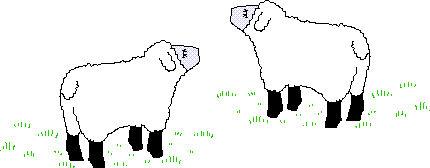#### You may also like### Room Doubling

Investigate the different ways you could split up these rooms so that you have double the number.### Fibonacci's Three Wishes 2

Second of two articles about Fibonacci, written for students.### Leonardo of Pisa and the Golden Rectangle

Leonardo who?! Well, Leonardo is better known as Fibonacci and this article will tell you some of fascinating things about his famous sequence.

# Sheep Talk

## Sheep TalkIn sheep talk the only letters used are B and A. Sequences of words are formed as follows:

The first word only contains the single letter A.

To get the next word in the sequence change each A in the previous word into B and each B in the previous word into AB.

Write down the first ten words in this sequence.

Count the number of A's in each word in the sequence. Then count the number of B's in each word in the sequence. Finally count the number of letters in each word in the sequence. You now have three sequences of numbers. What do you notice? Will the same patterns continue if you generate longer sequences of words?

Why do this problem?
Doing this investigation learners notice patterns and make and prove conjectures. It offers one of the best ways for learners to discover Fibonacci sequences for themselves. Whether or not they have already met these sequences it is important to ask whether the pattern will continue and, more importantly, why it will continue?

Learners will notice that the same sequences of numbers occur when they count the number of A's in the words, when they count the number of B's in the words and also when they count the total number of letters.The teacher could simply get the learners to count the letters, fill in a table, make conjectures about how many A's and B's in the next word and then check if their conjectures were correct. Then she can choose how long to pursue the reasons and the proof that the sequences are Fibonacci sequences.

For the proof of why this process gives the Fibonacci sequence you have to consider how the letters change from one word to the next and generate copies of themselves. This can lead to a lot of mathematical talk and it can give excellent practice in mathematical reasoning and communication.

Possible approach
As with all problem solving, the first step is to understand the question. If the teacher asks the learners to read the question for themselves and then to tell her the rule it will give the learners practice in thinking for themselves. Asking the learners to write the next word on their individual 'show-me boards' ensures that every learner is actively involved. A list of about six words should be written down and then a table of results set up and the numbers of letters filled in the table.

Learners can each fill in their own tables and be asked to work in pairs to see what patterns they can spot. When they see a pattern (conjecture) they should be asked to use the Sheep Rule to write down some more words in the sequence and see if the pattern continues (test the conjecture). If they find it does then learners should be asked to try to explain why the pattern comes out that way.

Learners can be asked to write down the rule for the sequence in words and then perhaps if they can use symbols to give the rule. At this age the teacher may simply accept the different notations offered by the class members or choose to discuss with the class why it is convenient to have an agreed (standard) notation. This could lead comfortably to using algebraic notation as only counting is involved and not solving equations, which is perhaps more demanding.

(The Fibonacci sequence can be defined as the sequence where the $n$th term is denoted by $F_n$, where $F_0$ and $F_1$ are $0$ or $1$ and $F_n=F_{n-1}+F_{n-2}$.)

Key questions
Do you notice a pattern in the sequence of numbers?
How many A's do you think there will be in the next word (that you have not written down yet)?
Would you like to write down the next word in the list and see if you were right?
Look at an A in a word. How many A's come from it in the next word and in the word after that?
How many A's in a word come from an AB in the word before it?
How do you find the number of A's in the tenth word? Why?
Would you have to write the whole list of 20 words to find out how many A's there would be in the twentieth word?